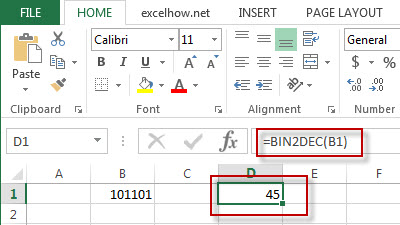## Excel Bin2Dec Function

This post will guide you how to use Excel BIN2DEC function with syntax and examples in Microsoft excel.

### Description

The Excel BIN2DEC function converts a binary number to decimal.

The BIN2DEC function is a build-in function in Microsoft Excel and it is categorized as an Engineering Function.

The BIN2DEC function is available in Excel 2016, Excel 2013, Excel 2010, Excel 2007, Excel 2003, Excel XP, Excel 2000, Excel 2011 for Mac.

### Syntax

The syntax of the BIN2DEC function is as below:

= BIN2DEC (binary_number)

Where the BIN2DEC function argument is:
Binary_number -This is a required argument. The binary number that you want to convert to decimal.

### Example

The below examples will show you how to use Excel BIN2DEC Function to convert a binary number to a decimal number.

#1 = BIN2DEC (B1)